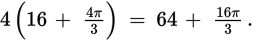# SAT Math Multiple Choice Question 387: Answer and Explanation

### Test Information

Question: 387

12. A rodeo is building a circular arena. The arena will have a total area of 64π square yards and can either be left open for rodeo competitions or divided into 12 equal sections through the center for auctions. When holding auctions, the rodeo has an average of 4 bulls and 8 horses for sale. A bull cannot be placed in a section directly beside another section containing a bull, and all edges of these sections must be reinforced with strong steel to keep the bulls from getting out. Which of the following represents how much steel in yards the rodeo will need to reinforce the four bull sections?

• A. 32π
• B. 64π
• C.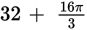• D.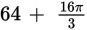Explanation:

D

Difficulty: Hard

Category: Additional Topics in Math / Geometry

Strategic Advice: Drawing a sketch is the key to answering this question.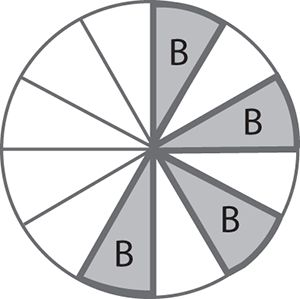Notice that two radii and an arc form each section, so the amount of steel needed for one section is the length of the radius times 2, plus the length of the outer arc. Once you know this, you can simply multiply by 4.

Getting to the Answer: You are given the total area, which means you can find the radius by substituting 64π for A in the area formula, A = πr 2.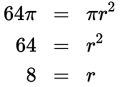For auctions, the arena is divided into 12 equal sections through the center, so divide 360 by 12 to find that the central angle measure for each section is 30°. Now use the arc length formula: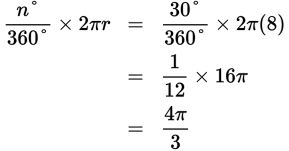The amount of steel needed for one section is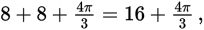so the amount needed for all four sections is